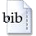User: GuestLogin
Document type:
Masterarbeit
Author(s):
Lukas Dreier
Title:
Identifiability of Cyclic Structural Equation Models with Gaussian Homoscedastic Error Terms
Abstract:
We prove that p-cycles in structural equation models with an equal Gaussian variance assumption are identifiable under a certain condition. The condition is formulated with regard to the coefficient matrix. The edge weights need to have a different absolute value to ensure identifiability of the structural equation model. The partial identifiability statement is proven by analyzing the structure of the precision matrix of the structural equation model. Partial identifiability implies that differ...    »
Subject:
MAT Mathematik
DDC:
510 Mathematik
Mathias Drton, Miguel de Benito Delgado
Date of acceptation:
31.03.2021
Year:
2021
Quarter:
3. Quartal
Year / month:
2021-03
Month:
Mar
Pages:
72
Language:
en
University:
Technische Universität München
Faculty:
Fakultät für Mathematik
TUM Institution:
Lehrstuhl für Mathematische Statistik
Format:
TextBibTeX